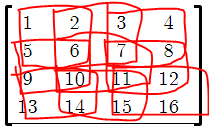# Explain CDRN02 problem

Contest is over can someone explain this https://www.codechef.com/COON2019/problems/CDRN02 problem.
for the test case provided i understood when A army fort is 3x3 and B army attacks like this in 2x2 .output : 4

if i considered B army is 2x2 again and the A army fort is 4x4.then the output would be 4 right ?

but why the answer is 9 i mean it would be like thisif this would be answer why the problem tester didnt provide another test case or explain it well if so many beginners like me would had understand .

The problem was not explained well at all.

you mean the question in the contest?